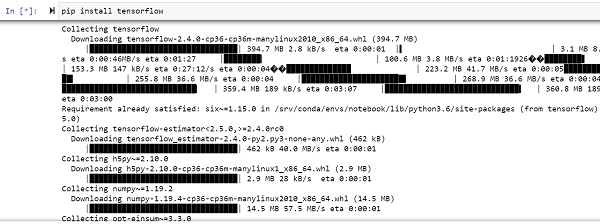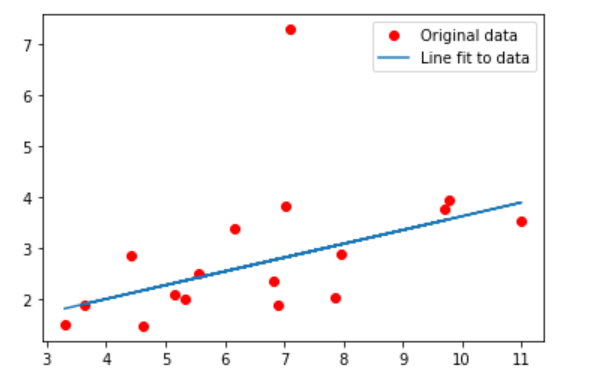# How can Linear Regression be implemented using TensorFlow?

Tensorflow is a machine learning framework that is provided by Google. It is an open−source framework used in conjunction with Python to implement algorithms, deep learning applications and much more. It is used in research and for production purposes.

The ‘tensorflow’ package can be installed on Windows using the below line of code −

pip install tensorflow

Tensor is a data structure used in TensorFlow. It helps connect edges in a flow diagram. This flow diagram is known as the ‘Data flow graph’. Tensors are nothing but multidimensional array or a list. They can be identified using three main attributes −

• Rank − It tells about the dimensionality of the tensor. It can be understood as the order of the tensor or the number of dimensions in the tensor that has been defined.

• Type − It tells about the data type associated with the elements of the Tensor. It can be a one dimensional, two dimensional or n dimensional tensor.

• Shape − It is the number of rows and columns together.

We will be using the Jupyter Notebook to run these codes. TensorFlow can be installed on Jupyter Notebook using ‘pip install tensorflow’.Following is an example −

## Example

from __future__ import absolute_import, division, print_function

import tensorflow as tf
import numpy as np
rng = np.random
print("The parameters have been randomly defined")
learning_rate = 0.02
training_steps = 1000
display_step = 100

X = np.array([3.3,4.43,5.15,6.17,6.9,4.62,9.70,6.81,7.95,3.63,
7.02,11.0,5.33,7.87,5.55,9.77,7.1])
Y = np.array([1.5,2.86,2.1,3.4,1.89,1.478,3.78,2.3456,2.89,1.874,
3.82,3.55,2.0,2.04,2.52,3.94,7.3])
print("The data has been generated")
A = tf.Variable(rng.randn(), name="weight")
b = tf.Variable(rng.randn(), name="bias")

def linear_reg(x):
return A * x + b
def mean_square_error(y_pred, y_true):
return tf.reduce_mean(tf.square(y_pred - y_true))
optimizer = tf.optimizers.SGD(learning_rate)

def run_optimization():
pred = linear_reg(X)
loss = mean_square_error(pred, Y)

print("The data is being trained")
for step in range(1, training_steps + 1):
run_optimization()
if step % display_step == 0:
pred = linear_reg(X)
loss = mean_square_error(pred, Y)
print("step: %i, loss: %f, W: %f, b: %f" % (step, loss, W.numpy(), b.numpy()))
print("The visualization of original data and data fit to model")
import matplotlib.pyplot as plt
plt.plot(X, Y, 'ro', label='Original data')
plt.plot(X, np.array(W * X + b), label='Line fit to data')
plt.legend()
plt.show()

## Output

The parameters have been randomly defined
The data has been generated
The data is being trained
step: 100, loss: 1.540684, W: 0.270616, b: 1.315416
step: 200, loss: 1.448993, W: 0.270616, b: 1.194412
step: 300, loss: 1.445010, W: 0.270616, b: 1.106874
step: 400, loss: 1.443114, W: 0.270616, b: 1.046157
step: 500, loss: 1.442205, W: 0.270616, b: 1.004092
step: 600, loss: 1.441768, W: 0.270616, b: 0.974950
step: 700, loss: 1.441558, W: 0.270616, b: 0.954762
step: 800, loss: 1.441458, W: 0.270616, b: 0.940776
step: 900, loss: 1.441409, W: 0.270616, b: 0.931087
step: 1000, loss: 1.441386, W: 0.270616, b: 0.924374
The visualization of original data and data fit to model## Explanation

• The required packages are imported and aliased.

• The learning parameters are defined. The training data is defined using the NumPy package.

• The ‘weight’ and ‘bias’ values are randomly initialized. They will be updated to optimal values once the training is complete.

• The general format for a linear equation is ‘Ax + b’ where ‘A’ is the ‘weight’ and ‘b’ is the ‘bias’ value.

• Function to calculate the mean square error is defined.

• The stochastic gradient descent optimizer is also defined.

• A function for optimization is defined, that computes gradients and updates the value of weights and bias.

• The data is trained for a specified number of steps.

• The built model is tested on the validation set.

• The predictions are visualized.